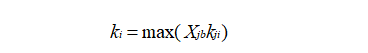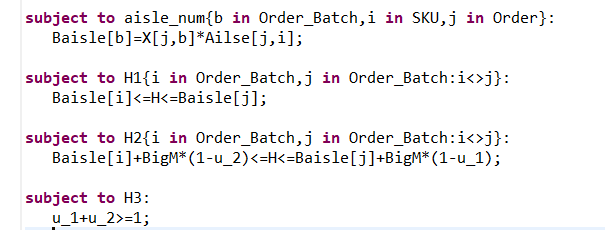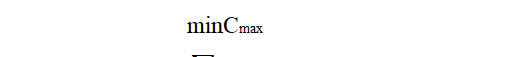# [AMPL 24618] AMPL Inquiries

Dear AMPL customer servicer：

Hello!I have some questions about AMPL I would like to ask you.My research problem is the problem of assembly shop scheduling.But I had trouble building models with AMPL.Here are my questions：
The first one，as shown in Figure 1, I want to ask for the maximum value in parentheses, whether there are any functions in AMPL that can directly find the maximum value, or if it needs to be linearized like Figure 2, and whether my linearization is correct …
Figure 1:Figure 2:Secondly, as shown in Figure 3, how these two constraints should be written in AMPL, should they also be linearized？

Figure 3:The lastly, as shown in Figure 4, my problem is the assembly shop scheduling problem, the final target value is to minimize the maximum completion time, this is calculated in the constraint, how to write this goal in AMPL?

Figure 4: